# Two cars

Two cars, spaced d1 = 500 m apart, start moving against each other at time t1 = 0, the first car with acceleration a1 = 4 m/s ^ 2 and the second with acceleration a2 = 6 m/s ^ 2. At what time t2 do they meet? What distances s1 and s2 will they move from the start to the moment of meeting both cars?

t2 =  10 s
s1 =  200 m
s2 =  300 m

### Step-by-step explanation: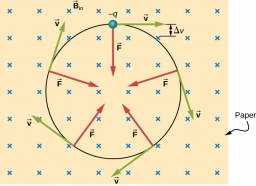Did you find an error or inaccuracy? Feel free to write us. Thank you!Tips to related online calculators
Do you want to convert length units?
Do you want to convert time units like minutes to seconds?

## Related math problems and questions:

• CollisionThe two bodies, whose initial distance is 240 m, move evenly against each other consistently. The first body has an initial velocity of 4 m/s and an acceleration of 3 m/s2, the second body has an initial speed of 6 m/s and an acceleration of 2 m/s2. Fin
• AccelerationThe car accelerates at a rate of 0.5 m/s2. How long travels 400 meters, and what will be its speed?
• Two cars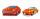From the town A to town B started two cars. The first at 7:00 at average speed 60 km per hour, the second at 10:00 at average speed 100 km per hour. The first car will not stay in B, and on the way back meet the second car at half way from A to B. At what
• Two carsTwo cars (trucks and passenger) drove simultaneously from two places 50 km away. If they go against each other, they will meet in 30 minutes. If they follow each other in the same direction, the car will catch up with the truck in 2.5 hours. What is the s
• Two friendsTwo friends on a bicycle rode against each other from two places 49 km apart. The first set off at 8.00 at a speed of 20km/h, the second 12 minutes later at a speed of 25km/h. What time do they meet? How many km will each of them travel since then?
• Cars 2Opava is 360 km distance from Prešov. At 8:00 hour, two cars started against each other from this cities at average speeds 88 km/h and 121 km/h. What hour will it meet and what distance it's travel if the car started from Prešov is faster?
• Against each other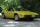From two points A, B distant 23 km at the same time started two cars against each other at speeds 41 km/h and 65 km/h. How long does cars meet and what distance passes each of them?
• Two cars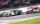Car A1 goes at average speed of 106 km/h and the second car A2 goes at 103 km/h. How many second will it take car A1 to circulate car A2? Assume that both cars are 5 meters long and the safety gap between cars is 1.5 meters.
• DriverThe car driver drives at a speed of 100 km/h, saw the obstacle, and began to brake with a slowing of 5 m/s². What is the length of the pathway to stopping the car when the driver has registered the obstacle with a delay of 0.7 s?
• Two carsTwo cars started against each other at the same time to journey long 346 km. The first car went 53 km/h and the second 53 km/h. What distance will be between these cars 20 minutes before meet?
• Brno-Tachov highwayThe distance Brno-Tachov is 360 km. At 8:00 a. M. , a car travels from Brno on the highway, traveling at an average speed of 90 km/h. An hour later, a car leaves Tachov towards Brno, whose average speed is 110 km/h. Calculate how far apart they will be at
• Cars speedsDistance from city A to city B is 108 km. Two cars were simultaneously started from both places. The speed of a car coming from city A was 2 km/h higher than the second car. What was the speed of each car if they met in 54 minutes?
• Fly and cyclist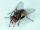Two cyclists are at 20 km apart on a same line. They start at same time towards each other at a speed of 10 km/hr. A fly sitting on one of the cyclists handle start flying towards the other cyclists at a speed of 20 km/hr. It touches the handle and move b
• Two airportsTwo airports are 2690 km away. From the first airport, the airplane flies at a speed of 600 km/h, from a second airplane at a speed of 780 km/h. When will they meet if they flew at 10:00? How far from the first airport?
• The positionThe position of a body at any time T is given by the displacement function S=t3-2t2-4t-8. Find its acceleration at each instant time when the velocity is zero.
• Motion against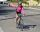From two places 36 km apart, a pedestrian and a cyclist will go straight opposite each other. How long will they meet if the speed of the pedestrian is 4 km/h and the cyclist 12 km/h?
• Cars motion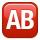From point A car start at the speed of 90 km/h. Against it departs from the point B at the same time another car at 60 km/h. For how long and where they met if the distance between A and B is 180 km?